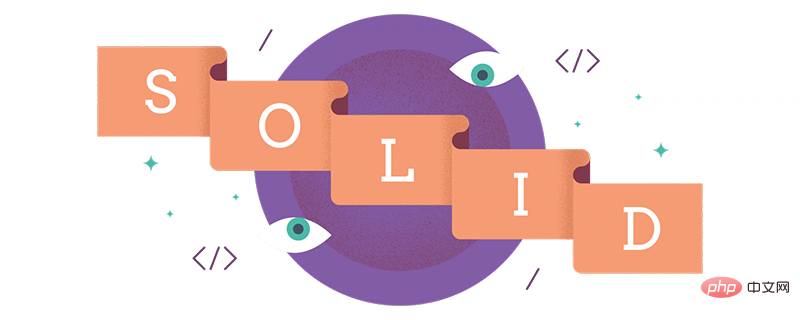PHP互联网工程师当前位置：主页 > 高端课程 > PHP互联网工程师 >

PHP 面向对象设计的五个基准原则S.O.L.I.D首个 5 个面向对象设计(OOD) 准则的首字母缩写 ，这些准则是由 Robert C. Martin 提出的, 他更为人所熟知的名字是 Uncle Bob。

• S - 单一功能原则
• O - 开闭原则
• L - 里氏替换原则
• I - 接口隔离原则
• D - 依赖反转原则

## 单一职责原则

```class Circle {
public \$radius;

public function construct(\$radius) {
\$this->radius = \$radius;
}
}

class Square {
public \$length;

public function construct(\$length) {
\$this->length = \$length;
}
}```

```class AreaCalculator {

protected \$shapes;

public function __construct(\$shapes = array()) {
\$this->shapes = \$shapes;
}

public function sum() {
// logic to sum the areas
}

public function output() {
return implode('', array(
"",
"Sum of the areas of provided shapes: ",
\$this->sum(),
""
));
}
}```

AreaCalculator 使用方法，我们只需简单的实例化这个类，并且传递一个图形数组，在页面底部展示输出内容．

```\$shapes = array(
new Circle(2),
new Square(5),
new Square(6)
);

\$areas = new AreaCalculator(\$shapes);

echo \$areas->output();```

SumCalculatorOutputter 类的工作方式如下：

```\$shapes = array(
new Circle(2),
new Square(5),
new Square(6)
);

\$areas = new AreaCalculator(\$shapes);
\$output = new SumCalculatorOutputter(\$areas);

echo \$output->JSON();
echo \$output->HAML();
echo \$output->HTML();
echo \$output->JADE();```

## 开闭原则

```public function sum() {
foreach(\$this->shapes as \$shape) {
if(is_a(\$shape, 'Square')) {
\$area[] = pow(\$shape->length, 2);
} else if(is_a(\$shape, 'Circle')) {
\$area[] = pi() * pow(\$shape->radius, 2);
}
}

return array_sum(\$area);
}```

```class Square {
public \$length;

public function __construct(\$length) {
\$this->length = \$length;
}

public function area() {
return pow(\$this->length, 2);
}
}```

```public function sum() {
foreach(\$this->shapes as \$shape) {
\$area[] = \$shape->area();
}

return array_sum(\$area);
}```

```interface ShapeInterface {
public function area();
}

class Circle implements ShapeInterface {
public \$radius;

public function __construct(\$radius) {
\$this->radius = \$radius;
}

public function area() {
return pi() * pow(\$this->radius, 2);
}
}```

```public function sum() {
foreach(\$this->shapes as \$shape) {
if(is_a(\$shape, 'ShapeInterface')) {
\$area[] = \$shape->area();
continue;
}

throw new AreaCalculatorInvalidShapeException;
}

return array_sum(\$area);
}```

## 里氏替换原则

```class VolumeCalculator extends AreaCalulator {
public function construct(\$shapes = array()) {
parent::construct(\$shapes);
}

public function sum() {
// logic to calculate the volumes and then return and array of output
return array(\$summedData);
}
}```

SumCalculatorOutputter 类:

```class SumCalculatorOutputter {
protected \$calculator;

public function __constructor(AreaCalculator \$calculator) {
\$this->calculator = \$calculator;
}

public function JSON() {
\$data = array(
'sum' => \$this->calculator->sum();
);

return json_encode(\$data);
}

public function HTML() {
return implode('', array(
'',
'Sum of the areas of provided shapes: ',
\$this->calculator->sum(),
''
));
}
}```

```\$areas = new AreaCalculator(\$shapes);
\$volumes = new AreaCalculator(\$solidShapes);

\$output = new SumCalculatorOutputter(\$areas);
\$output2 = new SumCalculatorOutputter(\$volumes);```

```public function sum() {
// logic to calculate the volumes and then return and array of output
return \$summedData;
}```

`\$summedData` 是一个浮点数、双精度浮点数或者整型。

## 接口隔离原则

```interface ShapeInterface {
public function area();
public function volume();
}```

ISP 原则不允许这么去做，所以我们应该创建另外一个拥有 `volume` 方法的`SolidShapeInterface` 接口去代替这种方式，这样类似立方体的实心体就可以实现这个接口了：

```interface ShapeInterface {
public function area();
}

interface SolidShapeInterface {
public function volume();
}

class Cuboid implements ShapeInterface, SolidShapeInterface {
public function area() {
//计算长方体的表面积
}

public function volume() {
// 计算长方体的体积
}
}```

```interface ManageShapeInterface {
public function calculate();
}

class Square implements ShapeInterface, ManageShapeInterface {
public function area() { /Do stuff here/ }

public function calculate() {
return \$this->area();
}
}

class Cuboid implements ShapeInterface, SolidShapeInterface, ManageShapeInterface {
public function area() { /Do stuff here/ }
public function volume() { /Do stuff here/ }

public function calculate() {
return \$this->area() + \$this->volume();
}
}```

## 依赖倒置原则

```class PasswordReminder {
private \$dbConnection;

public function __construct(MySQLConnection \$dbConnection) {
\$this->dbConnection = \$dbConnection;
}
}```

PasswordReminder 类不应该关注你的应用使用了什么数据库，为了进一步解决这个问题，我们「面向接口写代码」，由于高等级和低等级模块都应该依赖于抽象，我们可以创建一个接口：

```interface DBConnectionInterface {
public function connect();
}```

```class MySQLConnection implements DBConnectionInterface {
public function connect() {
return "Database connection";
}
}

class PasswordReminder {
private \$dbConnection;

public function __construct(DBConnectionInterface \$dbConnection) {
\$this->dbConnection = \$dbConnection;
}
}```

## 总结

• 全球最大晶圆代工半导体制造厂，台积电斥资订购艾斯摩尔机器设备
• 英特尔依然是那个英特尔，且看英特尔的城防体系
• 支持双 DRAM 内存接口，慧荣企业级 SSD 主控方案披露
• 在全球被反垄断罚款，冤！高通到底哪里得罪了欧盟？
• 强强联合！万业、微电子所和芯鑫共同打造全新半导体设备
• 复旦大学校长称：对于集成电路产业发展，大学应该主动担当
• 技术再升级！无锡中科芯攻克晶圆级再布线及晶圆级凸点制备关键技
• 聚力！万业企业设立集成电路装备集团，提供自主可控设备
• 德州仪器C2000微控制器增强连通性和控制性
• 英特尔打出降价策略，以免被竞争对手 AMD 打败？
• 贸易摩擦的闹剧没有赢家，苹果有勇气离开中国吗？
• 图像信号与视觉处理器的发展趋势
• 真干快消品定位方案班（第二期）火热开班
• Java集合 ArrayList原理及使用
• TDD（测试驱动开发）死了吗？
• JAVA基础之XML相关
• javaweb项目搭建ehcache缓存系统
• 每日一码——字符串统计
• 一篇文章帮你彻底搞清楚“I/O多路复用”和“异步I/O”的前世今生
• 九：模板方法模式
• 十二：命令模式（人员解耦和）
• Java 转PPT为图片、PDF、SVG、XPS、ODP以及PPT和PPTX互转
• SpringCloud学习（SPRINGCLOUD微服务实战）一
• 记一次微信网页授权后获取用户信息并重定向
• 速途新营销五点实战洞察解码“品效合一”
• 十一：外观模式详解（Service，action与dao）
• 手把手教你学会 基于JWT的单点登录
• mysql锁机制总结，以及优化建议
• 解决多个版本jar包冲突【jar内包名重命名】
• 中国首张5G终端电信设备进网许可证 华为Mate 20 X 5G版入网
• RPC之Thrift
• 高级Java工程师必备 ----
• 天猫618期间实物支付GMV增长38.5%
• 换季了，老板你的库存处理好了吗？
• 从“618”大数据看中国消费新活力
• 小米生态链：贵在格局感与收放度
• CODING 2.0 企业级持续交付解决方案
• 老铁奇趴“新京济” 快手*京东618战报出炉
• 中小企业新媒体运营基本技能
• 上汽大通房车再度携手LINE FRIENDS 魔都巡游顺利开启
• 华为高端手机国内市场份额超苹果夺得榜首
• 中国智能制造分析报告
• iPlus艾加营销助力腾讯广告牵手吴晓波 推进商业IP变现
• 2019世界新能源汽车大会7月1日将在海南举行
• 区域酒企如何转型突围
• 时时彩论坛
• 五星体育斯诺克
• 北单比分直播
• 河北11选5走势图
• 福建体彩36选7开奖结果
• 九龙图库下载